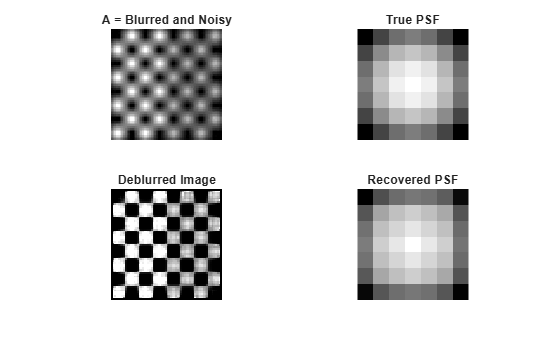# deconvblind

Deblur image using blind deconvolution

## Syntax

``[J,psfr] = deconvblind(I,psfi)``
``[J,psfr] = deconvblind(I,psfi,iter)``
``[J,psfr] = deconvblind(I,psfi,iter,dampar)``
``[J,psfr] = deconvblind(I,psfi,iter,dampar,weight)``
``[J,psfr] = deconvblind(I,psfi,iter,dampar,weight,readout)``
``[J,psfr] = deconvblind(___,fun)``

## Description

````[J,psfr] = deconvblind(I,psfi)` deconvolves image `I` using the maximum likelihood algorithm and an initial estimate of the point-spread function (PSF), `psfi`. The `deconvblind` function returns both the deblurred image `J` and a restored PSF, `psfr`.To improve the restoration, `deconvblind` supports several optional parameters, described below. Use `[]` as a placeholder if you do not specify an intermediate parameter.```
````[J,psfr] = deconvblind(I,psfi,iter)` specifies the number of iterations, `iter`.```
````[J,psfr] = deconvblind(I,psfi,iter,dampar)` controls noise amplification by suppressing iterations for pixels that deviate a small amount compared to the noise, specified by the damping threshold `dampar`. By default, no damping occurs.```

example

````[J,psfr] = deconvblind(I,psfi,iter,dampar,weight)` specifies which pixels in the input image `I` are considered in the restoration. The value of an element in the `weight` array determines how much the pixel at the corresponding position in the input image is considered. For example, to exclude a pixel from consideration, assign it a value of `0` in the `weight` array. You can adjust the weight value assigned to each pixel according to the amount of flat-field correction.```
````[J,psfr] = deconvblind(I,psfi,iter,dampar,weight,readout)` specifies the additive noise (such as background and foreground noise) and the variance of the read-out camera noise, `readout`.```
````[J,psfr] = deconvblind(___,fun)`, where `fun` is a handle to a function that describes additional constraints on the PSF. `fun` is called at the end of each iteration. For more information about function handles, see Create Function Handle.```

## Examples

collapse all

Create a sample image with noise.

```% Set the random number generator back to its default settings for % consistency in results. rng default; I = checkerboard(8); PSF = fspecial('gaussian',7,10); V = .0001; BlurredNoisy = imnoise(imfilter(I,PSF),'gaussian',0,V);```

Create a weight array to specify which pixels are included in processing.

```WT = zeros(size(I)); WT(5:end-4,5:end-4) = 1; INITPSF = ones(size(PSF));```

Perform blind deconvolution.

`[J P] = deconvblind(BlurredNoisy,INITPSF,20,10*sqrt(V),WT);`

Display the results.

```subplot(221);imshow(BlurredNoisy); title('A = Blurred and Noisy'); subplot(222);imshow(PSF,[]); title('True PSF'); subplot(223);imshow(J); title('Deblurred Image'); subplot(224);imshow(P,[]); title('Recovered PSF');```## Input Arguments

collapse all

Blurry image, specified as a numeric array of any dimension. You can also specify the image as a cell array to enable interrupted iterations. For more information, see Tips.

Data Types: `single` | `double` | `int16` | `uint8` | `uint16`

Initial estimate of PSF, specified as a numeric array. The PSF restoration is affected strongly by the size of the initial guess `psfi` and less by the values it contains. For this reason, specify an array of `1`s as your `psfi`.

You can also specify `psfi` as a cell array to enable interrupted iterations. For more information, see Tips.

Data Types: `single` | `double` | `int16` | `uint8` | `uint16`

Number of iterations, specified as a positive integer.

Data Types: `double`

Threshold for damping, specified as a numeric scalar. Damping occurs for pixels whose deviation between iterations is less than the threshold. `dampar` has the same data type as `I`.

Weight value of each pixel, specified as a numeric array with values in the range [0, 1]. `weight` has the same size as the input image, `I`. By default, all elements in `weight` have the value `1`, so all pixels are considered equally in the restoration.

Data Types: `double`

Noise, specified as a numeric scalar or numeric array. The value of `readout` corresponds to the additive noise (such as noise from the foreground and background) and the variance of the read-out camera noise. `readout` has the same data type as `I`.

Function handle, specified as a handle. `fun` must accept the PSF as its first argument. The function must return one argument: a PSF that is the same size as the original PSF and that satisfies the positivity and normalization constraints.

## Output Arguments

collapse all

Deblurred image, returned as a numeric array or a 1-by-4 cell array. `J` (or `J{1}` when `J` is a cell array) has the same data type as `I`. For more information about returning `J` as a cell array for interrupted iterations, see Tips.

Restored PSF, returned as an array of positive numbers or a 1-by-4 cell array. `psfr` has the same size as the initial estimate of the PSF, `psfi`, and it is normalized so the sum of elements is 1. For more information about returning `psfr` as a cell array for interrupted iterations, see Tips.

Data Types: `double`

## Tips

• You can use `deconvblind` to perform a deconvolution that starts where a previous deconvolution stopped. To use this feature, pass the input image `I` and the initial guess at the PSF, `psfi`, as cell arrays: `{I}` and `{psfi}`. When you do, the `deconvblind` function returns the output image `J` and the restored point-spread function, `psfr`, as cell arrays, which can then be passed as the input arrays into the next `deconvblind` call. The output cell array `J` contains four elements:

`J{1}` contains `I`, the original image.

`J{2}` contains the result of the last iteration.

`J{3}` contains the result of the next-to-last iteration.

`J{4}` is an array generated by the iterative algorithm.

• The output image `J` could exhibit ringing introduced by the discrete Fourier transform used in the algorithm. To reduce the ringing, use `I = edgetaper(I,psfi)` before calling `deconvblind`.

 D.S.C. Biggs and M. Andrews, Acceleration of iterative image restoration algorithms, Applied Optics, Vol. 36, No. 8, 1997.

 R.J. Hanisch, R.L. White, and R.L. Gilliland, Deconvolutions of Hubble Space Telescope Images and Spectra, Deconvolution of Images and Spectra, Ed. P.A. Jansson, 2nd ed., Academic Press, CA, 1997.

 Timothy J. Holmes, et al, Light Microscopic Images Reconstructed by Maximum Likelihood Deconvolution, Handbook of Biological Confocal Microscopy, Ed. James B. Pawley, Plenum Press, New York, 1995.